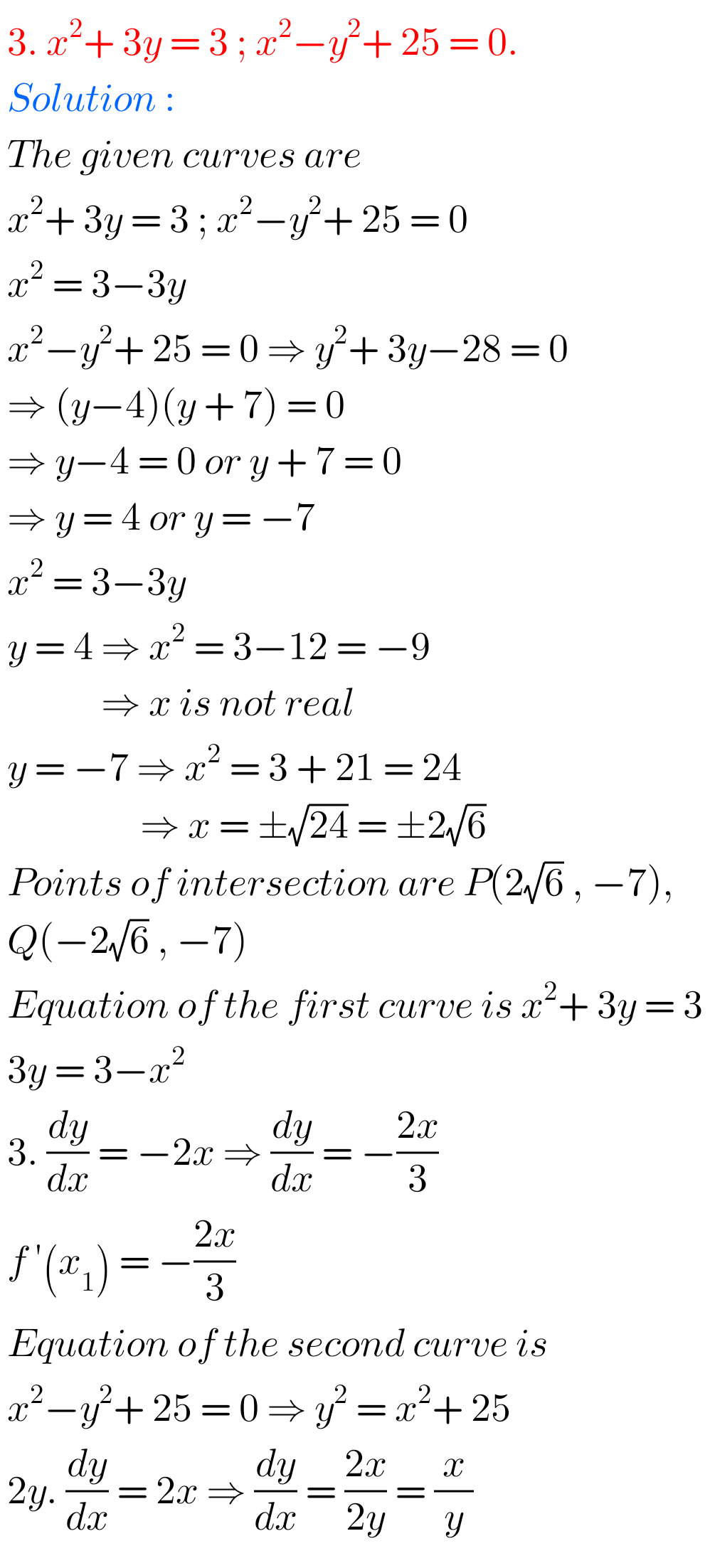## Applications of Derivatives exercise 10(d) solutions Inter

Inter Maths Solutions for Applications of Derivatives exercise 10(d) Intermediate Mathematices 1B Solutions for exercise 10(d) Applications of Derivatives are given. Study the textbook lesson Applications of Derivatives very well. Practice the example problems and solutions given in the textbook. Observe the solutions given below and try them in your own method. You can also …